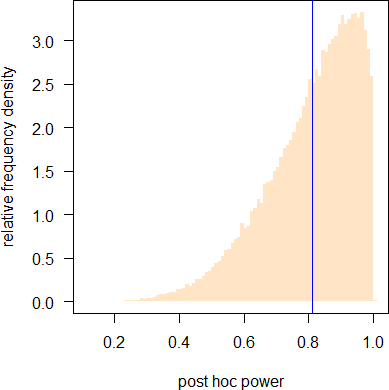## Yes, but why? [Power / Sample Size]

Hi kms,

❝ These are the results i'm finding maximum times.

THX for providing the data. I guess you mean maximum concentrations?
When doubting your calculations I stand corrected!

library(PowerTOST) # assumed CV.0     <- 0.2 # CV-intra theta0.0 <- 1.1 # T/R-ratio target   <- 0.8 # desired (target) power dor      <- 10  # expected dropout rate in percent # observed CV.1     <- 0.1836 theta0.1 <- 0.96 n        <- 34 # sample size and power plan     <- sampleN.TOST(CV=CV.0, theta0=theta0.0, targetpower=target) # Adjust for expected dropout rate and round up to next even N.ad     <- ceiling(plan[["Sample size"]]/(1-dor/100)/2)*2 # power for various number of dropouts # elig     <- seq(N.ad, 2*plan[["Sample size"]]-N.ad, -1) pwr1     <- data.frame(dosed=rep(N.ad, length(elig)),                        dropouts=N.ad-elig, eligible=elig, power=NA) for (j in seq_along(elig)) {   pwr1\$power[j] <- suppressMessages(power.TOST(CV=CV.0, theta0=theta0.0,                                                n=elig[j])) } cat("Ante hoc power for various number of dropouts:\n"); print(signif(pwr1, 4), row.names=FALSE) cat("Post hoc power:", signif(power.TOST(CV=CV.1, theta0=theta0.1, n=n), 4), "\n")

Gives:

+++++++++++ Equivalence test - TOST +++++++++++             Sample size estimation ----------------------------------------------- Study design:  2x2 crossover log-transformed data (multiplicative model) alpha = 0.05, target power = 0.8 BE margins = 0.8 ... 1.25 True ratio = 1.1,  CV = 0.2 Sample size (total)  n     power 32   0.810068

Ante hoc power for various number of dropouts:  dosed dropouts eligible  power     36        0       36 0.8505     36        1       35 0.8409     36        2       34 0.8314     36        3       33 0.8207     36        4       32 0.8101     36        5       31 0.7982     36        6       30 0.7864     36        7       29 0.7732     36        8       28 0.7601 Post hoc power: 0.9917 

Modifying my simulation code for theta0=1/1.1 and n=34 I got:
1e+05 simulated studies with “post hoc” power of   ≥ target    : 59.75%   ≥ achieved  : 57.18%   ≥ 0.90      : 31.51%   ≥ 0.95      : 15.18%   [0.95, 0.99]: 12.59%   ≥ 0.9917    :  2.11%Hence, such a high power is unlikely but possible.

As usual power is more sensitive to changes in the GMR than in the CV:
pwr.0 <- power.TOST(CV=0.2, theta0=1/1.1, n=34) cat("Relative increase in power due to",     "\n  better CV :", sprintf("%5.2f%%",     100*(power.TOST(CV=0.1836, theta0=1/1.1, n=34)-pwr.0)/pwr.0),     "\n  better GMR:", sprintf("%5.2f%%",     100*(power.TOST(CV=0.2, theta0=0.96, n=34)-pwr.0)/pwr.0),     "\n  both      :", sprintf("%5.2f%%",     100*(power.TOST(CV=0.1836, theta0=0.96, n=34)-pwr.0)/pwr.0), "\n") Relative increase in power due to   better CV :  6.16%   better GMR: 17.95%   both      : 19.28%

One question remains open:

❝ Can you explain to us why you performed a “posthoc analysis” at all? What did you want to achieve? To repeat ElMaestro:

❝ ❝ ❝ ❝ Try and ask yourself which question post-hoc power actually answers. Try and formulate it in a very specific sentence.Dif-tor heh smusma 🖖🏼 Довге життя Україна!Helmut SchützThe quality of responses received is directly proportional to the quality of the question asked. 🚮
Science QuotesIng. Helmut Schütz